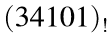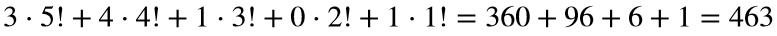# Problem 51560. Convert a number from factorial notation to base 10

Just as numbers can be written in different bases, they can also be written in factorial notation. For example,is 463 in base 10 becauseIf the first place after the decimal point is the 1/2! place, then we would have, for example,Write a function to convert a number from factorial notation to decimal notation, or base 10. For digits greater than 9, use A, B, C, etc.

### Solution Stats

50.0% Correct | 50.0% Incorrect
Last Solution submitted on Jun 17, 2023

### Community Treasure Hunt

Find the treasures in MATLAB Central and discover how the community can help you!

Start Hunting!